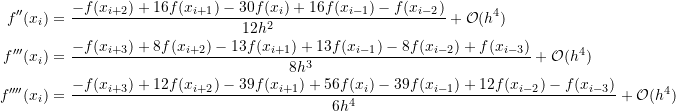### Numerical Differentiation: High-Accuracy Numerical Differentiation Formulas

### High-Accuracy Numerical Differentiation Formulas

The formulas presented in the previous sections for the forward and backward finite difference have an error term of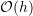while those for the centred finite difference scheme have an error term of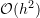. It is possible to provide formulas with less error by utilizing more terms in the Taylor approximation. In essence, by increasing the number of terms in the Taylor series approximation, we assume a higher-order polynomial for the approximation which increases the accuracy of the derivatives. In the following presentation, the function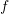is assumed to be smooth and the points are equidistant with a step size of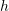.

#### Forward Finite Difference

According to Taylor theorem, we have: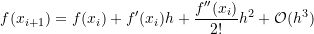Using the forward finite difference equation (Equation 3) for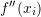yields: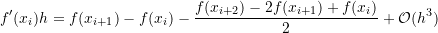Therefore: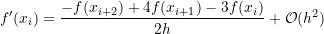In comparison with Equation 1, this equation provides an error term that is directly proportional to the square of the step size indicating higher accuracy.

Using the same procedure, the following equations can be obtained for the second, third, and fourth derivatives: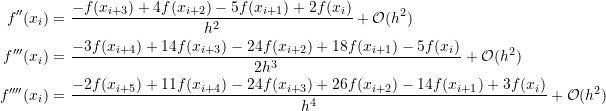#### Backward Finite Difference

According to Taylor theorem, we have: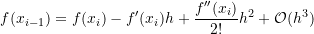Using the backward finite difference equation (Equation 4) foryields: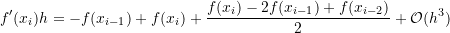Therefore: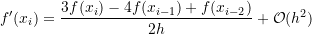In comparison with Equation 2, this equation provides an error term that is directly proportional to the square of the step size indicating higher accuracy.
Using the same procedure, the following equations can be obtained for the second, third, and fourth derivatives: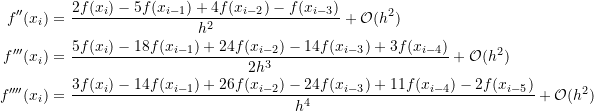#### Centred Finite Difference

According to Taylor theorem, we have: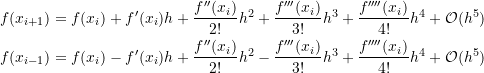Subtracting the above two equations and using the centred finite difference equation (Equation 6) for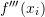yields: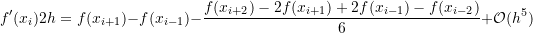Therefore: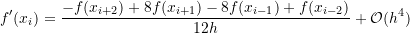Using the same procedure, the following equations can be obtained for the second, third, and fourth derivatives: